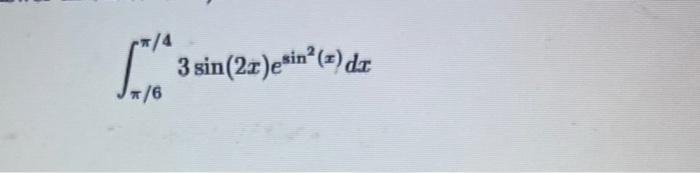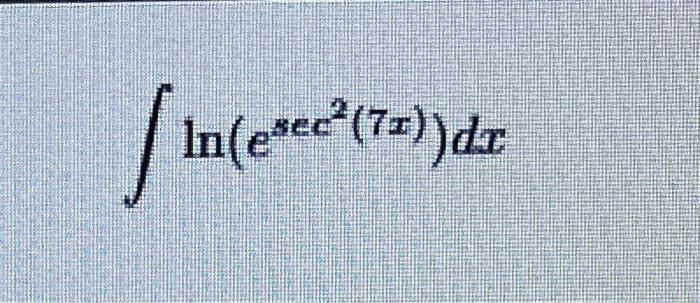Home / Expert Answers / Calculus / need-help-evaluating-the-integral-nbsp-int-pi-6-pi-4-3-sin-2-x-e-sin-2-x-d-pa170

# (Solved): need help evaluating the integral  $$\int_{\pi / 6}^{\pi / 4} 3 \sin (2 x) e^{\sin ^{2}(x)} d ... need help evaluating the integral\( \int_{\pi / 6}^{\pi / 4} 3 \sin (2 x) e^{\sin ^{2}(x)} d x$$ $$\int \ln \left(e^{\sec ^{2}(7 x)}\right) d x$$

We have an Answer from Expert